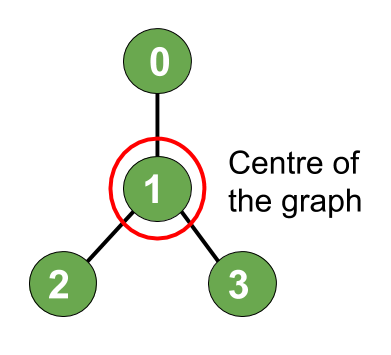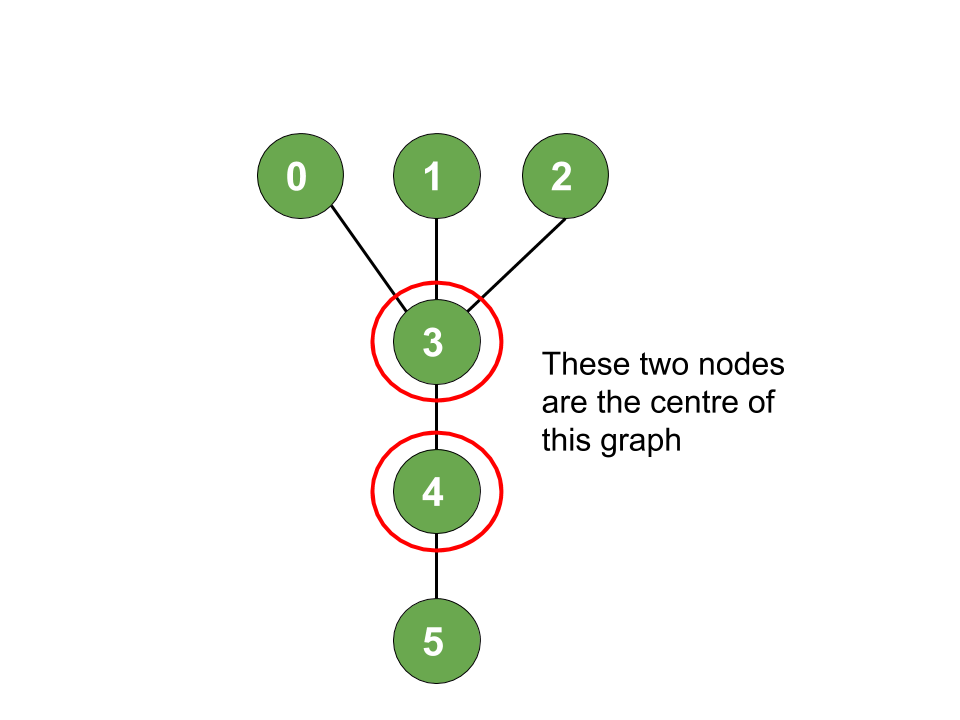Open in App
Not now

# Find the node at the center of an N-ary tree

• Difficulty Level : Expert
• Last Updated : 02 Dec, 2021

Prerequisites:

Given a N-ary tree with N nodes numbered from 0 to N-1 and a list of undirected edges, the task is to find the node(s) at the center of the given tree.

Eccentricity: The eccentricity of any vertex V in a given tree is the maximum distance between the given vertex V and any other vertex of the tree.
Center: The center of a tree is the vertex having the minimum eccentricity. Hence, it means that in order to find the center we have to minimize this eccentricity.

Examples:

Input: N = 4, Edges[] = { (1, 0), (1, 2), (1, 3)}
Output:
Explanation:Input: N = 6, Edges[] = { (0, 3), (1, 3), (2, 3), (4, 3), (5, 4)}
Output: 3, 4
Explanation:Approach: It can be observed that the path of maximum eccentricity is the diameter of the tree. Hence, the center of the tree diameter will be the center of the tree as well.

Proof:

• For example, Let’s consider a case where the longest path consists of odd number of vertices. Let the longest path be X —— O ——– Y where X and Y are the two endpoints of the path and O is the middle vertex.
• For a contradiction, if the center of the tree is not O but some other vertex O’, then at least one of the following two statements must be true.
1. Path XO’ is strictly longer than path XO
2. Path YO’ is strictly longer than path YO
• This means O’ will not satisfy the condition of minimum eccentricity. Hence by contradiction, we have proved that the center of the tree is actually the center of the diameter path.
• Now if the diameter consists odd number of nodes, then there exists only 1 center (also known as Central Tree).
• If diameter consists of even number of nodes, then there are 2 center nodes(also known as Bi-central Tree).

Below is the implementation of the above approach:

## C++

 `// C++ implementation of``// the above approach` `#include ``using` `namespace` `std;` `// To create tree``map<``int``, vector<``int``> > tree;` `// Function to store the path``// from given vertex to the target``// vertex in a vector path``bool` `getDiameterPath(``int` `vertex,``                     ``int` `targetVertex,``                     ``int` `parent,``                     ``vector<``int``>& path)``{` `    ``// If the target node is found,``    ``// push it into path vector``    ``if` `(vertex == targetVertex) {` `        ``path.push_back(vertex);``        ``return` `true``;``    ``}` `    ``for` `(``auto` `i : tree[vertex]) {` `        ``// To prevent visiting a``        ``// node already visited``        ``if` `(i == parent)``            ``continue``;` `        ``// Recursive call to the neighbours``        ``// of current node inorder``        ``// to get the path``        ``if` `(getDiameterPath(i, targetVertex,``                            ``vertex, path)) {``            ``path.push_back(vertex);``            ``return` `true``;``        ``}``    ``}` `    ``return` `false``;``}` `// Function to obtain and return the``// farthest node from a given vertex``void` `farthestNode(``int` `vertex, ``int` `parent,``                  ``int` `height, ``int``& maxHeight,``                  ``int``& maxHeightNode)``{` `    ``// If the current height is maximum``    ``// so far, then save the current node``    ``if` `(height > maxHeight) {``        ``maxHeight = height;``        ``maxHeightNode = vertex;``    ``}` `    ``// Iterate over all the neighbours``    ``// of current node``    ``for` `(``auto` `i : tree[vertex]) {``        ``// This is to prevent visiting``        ``// a already visited node``        ``if` `(i == parent)``            ``continue``;` `        ``// Next call will be at 1 height``        ``// higher than our current height``        ``farthestNode(i, vertex,``                     ``height + 1,``                     ``maxHeight,``                     ``maxHeightNode);``    ``}``}` `// Function to add edges``void` `addedge(``int` `a, ``int` `b)``{``    ``tree[a].push_back(b);``    ``tree[b].push_back(a);``}` `void` `FindCenter(``int` `n)``{``    ``// Now we will find the 1st farthest``    ``// node from 0(any arbitrary node)` `    ``// Perform DFS from 0 and update``    ``// the maxHeightNode to obtain``    ``// the farthest node from 0` `    ``// Reset to -1``    ``int` `maxHeight = -1;` `    ``// Reset to -1``    ``int` `maxHeightNode = -1;` `    ``farthestNode(0, -1, 0, maxHeight,``                 ``maxHeightNode);` `    ``// Stores one end of the diameter``    ``int` `leaf1 = maxHeightNode;` `    ``// Similarly the other end of``    ``// the diameter` `    ``// Reset the maxHeight``    ``maxHeight = -1;``    ``farthestNode(maxHeightNode,``                 ``-1, 0, maxHeight,``                 ``maxHeightNode);` `    ``// Stores the second end``    ``// of the diameter``    ``int` `leaf2 = maxHeightNode;` `    ``// Store the diameter into``    ``// the vector path``    ``vector<``int``> path;` `    ``// Diameter is equal to the``    ``// path between the two farthest``    ``// nodes leaf1 and leaf2``    ``getDiameterPath(leaf1, leaf2,``                    ``-1, path);` `    ``int` `pathSize = path.size();` `    ``if` `(pathSize % 2) {``        ``cout << path[pathSize / 2]``             ``<< endl;``    ``}``    ``else` `{``        ``cout << path[pathSize / 2]``             ``<< ``", "``             ``<< path[(pathSize - 1) / 2]``             ``<< endl;``    ``}``}` `// Driver Code``int` `main()``{` `    ``int` `N = 4;``    ``addedge(1, 0);``    ``addedge(1, 2);``    ``addedge(1, 3);` `    ``FindCenter(N);` `    ``return` `0;``}`

## Java

 `// Java implementation of``// the above approach``import` `java.util.*;` `class` `GFG{` `// To create tree``static` `Map> tree;``static` `ArrayList path;``static` `int` `maxHeight, maxHeightNode;` `// Function to store the path``// from given vertex to the target``// vertex in a vector path``static` `boolean` `getDiameterPath(``int` `vertex,``                               ``int` `targetVertex,``                               ``int` `parent,``                               ``ArrayList path)``{` `    ``// If the target node is found,``    ``// push it into path vector``    ``if` `(vertex == targetVertex)``    ``{``        ``path.add(vertex);``        ``return` `true``;``    ``}` `    ``for``(Integer i : tree.get(vertex))``    ``{``        ` `        ``// To prevent visiting a``        ``// node already visited``        ``if` `(i == parent)``            ``continue``;` `        ``// Recursive call to the neighbours``        ``// of current node inorder``        ``// to get the path``        ``if` `(getDiameterPath(i, targetVertex,``                            ``vertex, path))``        ``{``            ``path.add(vertex);``            ``return` `true``;``        ``}``    ``}``    ``return` `false``;``}` `// Function to obtain and return the``// farthest node from a given vertex``static` `void` `farthestNode(``int` `vertex, ``int` `parent,``                         ``int` `height)``{``    ` `    ``// If the current height is maximum``    ``// so far, then save the current node``    ``if` `(height > maxHeight)``    ``{``        ``maxHeight = height;``        ``maxHeightNode = vertex;``    ``}` `    ``// Iterate over all the neighbours``    ``// of current node``    ``if` `(tree.get(vertex) != ``null``)``        ``for``(Integer i : tree.get(vertex))``        ``{``            ` `            ``// This is to prevent visiting``            ``// a already visited node``            ``if` `(i == parent)``                ``continue``;` `            ``// Next call will be at 1 height``            ``// higher than our current height``            ``farthestNode(i, vertex,``                         ``height + ``1``);``        ``}``}` `// Function to add edges``static` `void` `addedge(``int` `a, ``int` `b)``{``    ``if` `(tree.get(a) == ``null``)``        ``tree.put(a, ``new` `ArrayList<>());` `    ``tree.get(a).add(b);` `    ``if` `(tree.get(b) == ``null``)``        ``tree.put(b, ``new` `ArrayList<>());` `    ``tree.get(b).add(a);``}` `static` `void` `FindCenter(``int` `n)``{``    ` `    ``// Now we will find the 1st farthest``    ``// node from 0(any arbitrary node)` `    ``// Perform DFS from 0 and update``    ``// the maxHeightNode to obtain``    ``// the farthest node from 0` `    ``// Reset to -1``    ``maxHeight = -``1``;` `    ``// Reset to -1``    ``maxHeightNode = -``1``;` `    ``farthestNode(``0``, -``1``, ``0``);` `    ``// Stores one end of the diameter``    ``int` `leaf1 = maxHeightNode;` `    ``// Similarly the other end of``    ``// the diameter` `    ``// Reset the maxHeight``    ``maxHeight = -``1``;``    ``farthestNode(maxHeightNode,``                 ``-``1``, ``0``);` `    ``// Stores the second end``    ``// of the diameter``    ``int` `leaf2 = maxHeightNode;` `    ``// Store the diameter into``    ``// the vector path``    ``path = ``new` `ArrayList<>();` `    ``// Diameter is equal to the``    ``// path between the two farthest``    ``// nodes leaf1 and leaf2``    ``getDiameterPath(leaf1, leaf2,``                    ``-``1``, path);` `    ``int` `pathSize = path.size();` `    ``if` `(pathSize % ``2` `== ``1``)``    ``{``        ``System.out.println(path.get(pathSize / ``2``));``    ``}``    ``else` `{``        ``System.out.println(path.get(pathSize / ``2``) +``                    ``", "` `+ path.get((pathSize - ``1``) / ``2``));``    ``}``}` `// Driver code``public` `static` `void` `main(String[] args)``{``    ``int` `N = ``4``;` `    ``tree = ``new` `HashMap<>();``    ``addedge(``1``, ``0``);``    ``addedge(``1``, ``2``);``    ``addedge(``1``, ``3``);` `    ``FindCenter(N);``}``}` `// This code is contributed by offbeat`

## Python3

 `# Python3 implementation of the above approach` `# To create tree``tree ``=` `{}``path ``=` `[]``maxHeight, maxHeightNode ``=` `-``1``, ``-``1` `# Function to store the path``# from given vertex to the target``# vertex in a vector path``def` `getDiameterPath(vertex, targetVertex, parent, path):``  ` `    ``# If the target node is found,``    ``# push it into path vector``    ``if` `(vertex ``=``=` `targetVertex):``        ``path.append(vertex)``        ``return` `True` `    ``for` `i ``in` `range``(``len``(tree[vertex])):``        ``# To prevent visiting a``        ``# node already visited``        ``if` `(tree[vertex][i] ``=``=` `parent):``            ``continue` `        ``# Recursive call to the neighbours``        ``# of current node inorder``        ``# to get the path``        ``if` `(getDiameterPath(tree[vertex][i], targetVertex, vertex, path)):``            ``path.append(vertex)``            ``return` `True``    ``return` `False` `# Function to obtain and return the``# farthest node from a given vertex``def` `farthestNode(vertex, parent, height):``    ``global` `maxHeight, maxHeightNode``    ``# If the current height is maximum``    ``# so far, then save the current node``    ``if` `(height > maxHeight):``        ``maxHeight ``=` `height``        ``maxHeightNode ``=` `vertex` `    ``# Iterate over all the neighbours``    ``# of current node``    ``if` `(vertex ``in` `tree):``        ``for` `i ``in` `range``(``len``(tree[vertex])):``          ` `            ``# This is to prevent visiting``            ``# a already visited node``            ``if` `(tree[vertex][i] ``=``=` `parent):``                ``continue``                ` `            ``# Next call will be at 1 height``            ``# higher than our current height``            ``farthestNode(tree[vertex][i], vertex, height ``+` `1``)` `# Function to add edges``def` `addedge(a, b):``    ``if` `(a ``not` `in` `tree):``        ``tree[a] ``=` `[]` `    ``tree[a].append(b)` `    ``if` `(b ``not` `in` `tree):``        ``tree[b] ``=` `[]` `    ``tree[b].append(a)` `def` `FindCenter(n):``    ``# Now we will find the 1st farthest``    ``# node from 0(any arbitrary node)` `    ``# Perform DFS from 0 and update``    ``# the maxHeightNode to obtain``    ``# the farthest node from 0` `    ``# Reset to -1``    ``maxHeight ``=` `-``1` `    ``# Reset to -1``    ``maxHeightNode ``=` `-``1` `    ``farthestNode(``0``, ``-``1``, ``0``)` `    ``# Stores one end of the diameter``    ``leaf1 ``=` `maxHeightNode` `    ``# Similarly the other end of``    ``# the diameter` `    ``# Reset the maxHeight``    ``maxHeight ``=` `-``1``    ``farthestNode(maxHeightNode, ``-``1``, ``0``)` `    ``# Stores the second end``    ``# of the diameter``    ``leaf2 ``=` `maxHeightNode` `    ``# Store the diameter into``    ``# the vector path``    ``path ``=` `[]` `    ``# Diameter is equal to the``    ``# path between the two farthest``    ``# nodes leaf1 and leaf2``    ``getDiameterPath(leaf1, leaf2, ``-``1``, path)` `    ``pathSize ``=` `len``(path)` `    ``if` `(pathSize ``%` `2` `=``=` `1``):``        ``print``(path[``int``(pathSize ``/` `2``)]``*``-``1``)``    ``else``:``        ``print``(path[``int``(pathSize ``/` `2``)], ``", "``, path[``int``((pathSize ``-` `1``) ``/` `2``)], sep ``=` `"``", end = "``")` `N ``=` `4``  ` `tree ``=` `{}``addedge(``1``, ``0``)``addedge(``1``, ``2``)``addedge(``1``, ``3``)` `FindCenter(N)` `# This code is contributed by suresh07.`

## C#

 `// C# implementation of``// the above approach``using` `System;``using` `System.Collections.Generic;``class` `GFG {``    ` `    ``// To create tree``    ``static` `Dictionary<``int``, List<``int``>> tree;``    ``static` `List<``int``> path;``    ``static` `int` `maxHeight, maxHeightNode;``     ` `    ``// Function to store the path``    ``// from given vertex to the target``    ``// vertex in a vector path``    ``static` `bool` `getDiameterPath(``int` `vertex,``                                   ``int` `targetVertex,``                                   ``int` `parent,``                                   ``List<``int``> path)``    ``{``     ` `        ``// If the target node is found,``        ``// push it into path vector``        ``if` `(vertex == targetVertex)``        ``{``            ``path.Add(vertex);``            ``return` `true``;``        ``}``     ` `        ``foreach``(``int` `i ``in` `tree[vertex])``        ``{``             ` `            ``// To prevent visiting a``            ``// node already visited``            ``if` `(i == parent)``                ``continue``;``     ` `            ``// Recursive call to the neighbours``            ``// of current node inorder``            ``// to get the path``            ``if` `(getDiameterPath(i, targetVertex,``                                ``vertex, path))``            ``{``                ``path.Add(vertex);``                ``return` `true``;``            ``}``        ``}``        ``return` `false``;``    ``}``     ` `    ``// Function to obtain and return the``    ``// farthest node from a given vertex``    ``static` `void` `farthestNode(``int` `vertex, ``int` `parent,``                             ``int` `height)``    ``{``         ` `        ``// If the current height is maximum``        ``// so far, then save the current node``        ``if` `(height > maxHeight)``        ``{``            ``maxHeight = height;``            ``maxHeightNode = vertex;``        ``}``     ` `        ``// Iterate over all the neighbours``        ``// of current node``        ``if` `(tree.ContainsKey(vertex) && tree[vertex].Count > 0)``        ``{``            ``foreach``(``int` `i ``in` `tree[vertex])``            ``{``                 ` `                ``// This is to prevent visiting``                ``// a already visited node``                ``if` `(i == parent)``                    ``continue``;``     ` `                ``// Next call will be at 1 height``                ``// higher than our current height``                ``farthestNode(i, vertex, height + 1);``            ``}``        ``}``    ``}``     ` `    ``// Function to add edges``    ``static` `void` `addedge(``int` `a, ``int` `b)``    ``{``        ``if` `(!tree.ContainsKey(a))``            ``tree[a] = ``new` `List<``int``>();``     ` `        ``tree[a].Add(b);``     ` `        ``if` `(!tree.ContainsKey(b))``            ``tree[b] = ``new` `List<``int``>();``     ` `        ``tree[b].Add(a);``    ``}``     ` `    ``static` `void` `FindCenter(``int` `n)``    ``{``         ` `        ``// Now we will find the 1st farthest``        ``// node from 0(any arbitrary node)``     ` `        ``// Perform DFS from 0 and update``        ``// the maxHeightNode to obtain``        ``// the farthest node from 0``     ` `        ``// Reset to -1``        ``maxHeight = -1;``     ` `        ``// Reset to -1``        ``maxHeightNode = -1;``     ` `        ``farthestNode(0, -1, 0);``     ` `        ``// Stores one end of the diameter``        ``int` `leaf1 = maxHeightNode;``     ` `        ``// Similarly the other end of``        ``// the diameter``     ` `        ``// Reset the maxHeight``        ``maxHeight = -1;``        ``farthestNode(maxHeightNode,``                     ``-1, 0);``     ` `        ``// Stores the second end``        ``// of the diameter``        ``int` `leaf2 = maxHeightNode;``     ` `        ``// Store the diameter into``        ``// the vector path``        ``path = ``new` `List<``int``>();``     ` `        ``// Diameter is equal to the``        ``// path between the two farthest``        ``// nodes leaf1 and leaf2``        ``getDiameterPath(leaf1, leaf2,``                        ``-1, path);``     ` `        ``int` `pathSize = path.Count;``     ` `        ``if` `(pathSize % 2 == 1)``        ``{``            ``Console.WriteLine(path[pathSize / 2]);``        ``}``        ``else` `{``            ``Console.WriteLine(path[pathSize / 2] +``                        ``", "` `+ path[(pathSize - 1) / 2]);``        ``}``    ``}` `  ``static` `void` `Main() {``    ``int` `N = 4;`` ` `    ``tree = ``new` `Dictionary<``int``, List<``int``>>();``    ``addedge(1, 0);``    ``addedge(1, 2);``    ``addedge(1, 3);`` ` `    ``FindCenter(N);``  ``}``}` `// This code is contributed by divyesh072019.`

## Javascript

 ``

Output:

`1`

Time Complexity: O(N)
Auxiliary Space: O(N)

My Personal Notes arrow_drop_up